A Note on the Interrelation of Volatility Puzzle, Equity Premium Puzzle, and Mean Reversion through State Dependent Preferences | OMICS International
Journal of Business & Financial Affairs

# A Note on the Interrelation of Volatility Puzzle, Equity Premium Puzzle, and Mean Reversion through State Dependent Preferences

Sung-Sup C1 and Giannikos IC2

2Department of Economics and Finance, Zicklin School of Business, Baruch College, City University of New York ,USA

*Corresponding Author:
Christos I Giannikos
Department of Economics and Finance
Baruch College/CUNY, One Baruch Way
Box B10-225, New York, USA
E-mail: [email protected]

Received June 26, 2013; Accepted September 17, 2013; Published Sptember 20, 2013

Citation: Sung-Sup C, Giannikos IC (2013) A Note on the Interrelation of Volatility Puzzle, Equity Premium Puzzle, and Mean Reversion through State Dependent Preferences. J Bus Fin Aff 2:112. doi:10.4172/2167-0234.1000112

Copyright: © 2013 Sung-Sup C, et al. This is an open-access article distributed under the terms of the Creative Commons Attribution License, which permits unrestricted use, distribution, and reproduction in any medium, provided the original author and source are credited.

Visit for more related articles at Journal of Business & Financial Affairs

#### Abstract

According to empirical studies, there is a systematic pattern in the temporal behavior of asset returns and this is related to the business cycle. We propose a simple model that captures this behavior. This model is built around a state dependent preference structure where the state dependency is related to the business cycle. In this setting the volatility puzzle, the equity premium puzzle and mean-reversion appear to be indeed interrelated phenomena. A necessary condition for the three puzzles to be explained is that the state variable is negatively correlated with the market portfolio cum business cycle.

#### Keywords

Volatility puzzle; Equity premium puzzle; Mean reversion

#### Introduction

In the standard utility-based asset-pricing model , a rational agent maximizes expected utility where preferences are assumed to be time-separable and state independent. It is a well-known fact that this model exhibits lack of volatility. It is indeed obvious that, in the absence of exogenous stochastic shocks, the system tends to a fixed point, which implies low volatility. Even in the case of exogenous shocks the resulting dynamics are more stable than empirical evidence suggests as Mehra and Prescott  have, among the first, demonstrated within the framework of an elegantly simple model. Among the various empirical studies that have verified the above Shiller , Hansen and Singleton  and Grossman, et al.  are quite prominent.

Thus, an improved asset-pricing model needs an ingredient, which can counteract the smoothing mechanism inherent in the classic model. Several approaches have been presented in attempting to construct models, which can overcome this deficiency. One approach by De Long et al.  can be viewed as an attempt to introduce some sort of irrationality. The form of irrationality chosen in this particular model requires the agent to be shortsighted and then more variability results. Another approach, by Epstein and Zin , has the agent more concerned about the timing of the resolution of uncertainty. This frustrates the intertemporal arbitrage operation, and thus distorts the smoothing mechanism. Yet another modeling approach, used initially by Constantinides , uses the idea of habit formation on the time domain. A positive feedback loop arises from this formulation, which uses a modified preference structure. This approach was extended by a series of papers including Campbell and Cochrane , who presented a precisely calibrated model that is broadly consistent with the stylized facts of the financial markets. In all of these different attempts, the common theme is to be able to disable the smoothing mechanism inherent in the dynamic structure of the standard model.

There have been a number of empirical studies though, which have been pointing out towards a different research route. For instance, Ferson and Merrick  found that the failure of the standard model is associated with the business cycle. By controlling this cyclical effect, they found less evidence against the standard model in non-recessionary periods but more evidence in recessionary periods. Schwert  related stock market volatility to some macro-economic variables, found correlations, and also revealed that both macro-economic variables and the stock market are more volatile in recessionary periods. Others have found that mean reversion in asset returns is related to stock market volatility Campbell and Shiller , for instance, claim that the mean reversion and volatility puzzle are interrelated phenomena), and the effect seems more significant in recessionary periods Schwert .

Overall there seems to be a cyclical pattern, related to the business cycle, in the observed temporal behavior of asset returns. Thus one could argue that the temporal behavior of asset returns is state dependent, where the state dependency arises from, and is related to, the business cycle. But the temporal behavior of asset returns should be explained within an asset-pricing model. This suggests that the inclusion of some state variable, which is correlated with the business cycle, should be a part of an alternative asset-pricing model.

We propose a simple model, which captures this idea. In this model, the investor’s preference structure, which includes a state variable, is correlated with the business cycle. Since the proposed preference is state dependent, the resulting dynamics will be more volatile and will blunt the smoothing mechanism inherent in the classical model. In addition to offering insights with regard to the volatility puzzle, the correlation structure in this model becomes a driving force that resolves both the equity premium puzzle and explains the mean reversion of asset prices. An interesting finding is that all three puzzles are, in this model, driven by the same correlation structure, which seems to suggest that the three are in fact interrelated phenomena.

The state dependent preferences recent literature includes Mehra and Sah , Kraus and Sagi , Melino and Yang  and Danthine et al. . Mehra and Sah  derive the partial equilibrium effects of small fluctuations in agent’s subjective discount rate and risk aversion on the volatility of asset prices. The other three papers undertake different variations of state-dependent risk aversion. Kraus and Sagi  analyze a complex multi agent model in continuous time where agents exhibit randomly changing risk aversion. Danthine et al.  employ the simplest variant of the standard model towards state dependency by allowing the representative agent’s coefficient of relative risk aversion to vary with the underlying economy’s growth rate. They demonstrate that for very low coefficients of relative risk aversion, the equilibrium risk free and risky security returns are demonstrated to have volatilities and an associated equity premium that substantially exceed what is found in data. Melino and Yang  undertake a similar exercise using Epstein-Zin/Weil preferences where the Coefficient of Relative Risk Aversion (CRRA) and the Elasticity of Intertemporal Substitution (EIS) can be specified independently. With state dependence in both CRRA and EIS, they are able to match perfectly the first two moments of equity and risk free returns. Finally Gordon and St-Amour  present a model of time varying risk aversion and estimate the implied process on risk aversion arising from consumption and financial data. They find that risk aversion is strongly countercyclical, rising during recessions and falling during expansions.

The outline of this paper is as follows: Section 2 presents the model and basic results, section 3 discusses the implications of the results and section 4 concludes.

#### The Model

This is a representative agent type of an exchange economy and can be thought of as an economy populated by many identical agents. There exists a single consumption good and two financial assets, a risky stock and a risk-free bond. It is an exchange economy and production and technology are not modeled here.

The rate of return of the risky asset follows the Ito process,(1)

with expected return a, volatility σP2P, and dz is a Wiener process.

The rate of return of the risk-free asset is r.

The equity, or risk, premium is naturally defined as,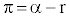(2)

The instantaneous direct utility function of the representative agent is u(c, x) where c is consumption and x is a state variable that follows an Ito process with mean f and standard deviation g,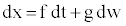(3)

where f and g are positive constants and w is a Wiener process.

If W is the wealth of the agent and wis the fraction invested in the risky asset then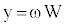(4)

is the amount of wealth invested in the risky asset.

Finally the correlation between the two Wiener processes dz and dw is given by,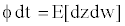(5)

Following is the optimization problem that the representative agent faces,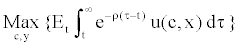(6)

subject to (3) and the following additional budget constraint: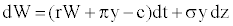(7)

Applying the Hamilton/Jacobi/Bellman equation and Ito’s lemma, the indirect utility function V (W, x) will satisfy the following equation,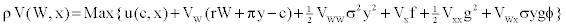(8)

where subscripts denote partial derivatives with respect to corresponding variables.

The first order conditions are,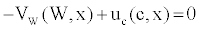(9)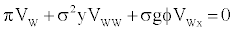(10)

In equilibrium, an investor holds all of his wealth in the risky asset, and net borrowing will be zero. Thus the equilibrium condition is,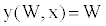(11)

In order to get a closed form solution we need one more refinement: to specify the instantaneous utility function. We assume that,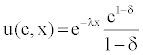(12)

where δ is the coefficient of the relative risk aversion (CRRA) and λ is a constant.

Under the above assumptions the value function should be of the following form: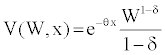(13)

With equation (12), equation (9) becomes,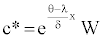(14)

From the above follows that,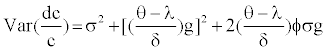(15)

Where σP2P= Var (dW/W), and φσg=Cov (dW/W, dx).

In equation (15), the first two terms of the consumption volatility are always positive. For the volatility of consumption to be less than that of wealth, the covariance (last) term has to be negative and must exceed the second term in absolute value. This requires, φ, the correlation between dx and dW, to be negative, assuming θ>λ. In other words, the negative correlation structure between dx and dW is a necessary condition for solving the volatility puzzle. In addition, if -2σφ>[(θ-λ)/δ] g and θ>xλ, the volatility of consumption is less than that of wealth.

Given equation (12), the portfolio choice equation (10) along with equilibrium condition (11) becomes,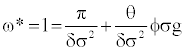(16)

where φσg=Cov (dW/W, x). In order for a high equity premium to be consistent with low RRA, the covariance term on the right-handside of equation (16) has to be negative. This requires the correlation between x and W to be negative, assuming θ>0. A solution to the equity premium puzzle, therefore, must satisfy φ<0, where θ>0. The reason for this is obvious since the equivalent equation for the standard model is missing the second term on the right-hand-side i.e. it is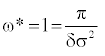(17)

With equation (12) and equation (13), we have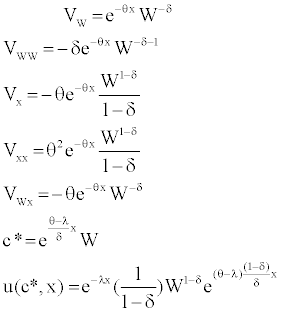(18)

Substituting (9), (10) and (11) into (8) yields,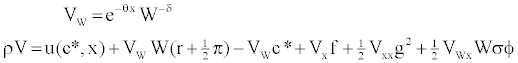(19)

Substituting (18) into (19), we get,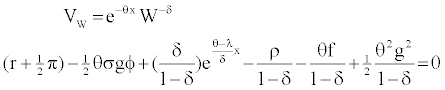(20)

Differentiating equation (20) with respect to x, we have the following,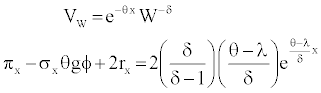(21)

We assume here that σBxB and rBxB are both equal to zero. The justification for this is the following. In regards to the interest rate derivative this is a direct consequence of the interest rate been easier to measure than the premium. Thus investors will respond more to interest rate changes than to premium changes. Their responses, in turn, will dampen the interest rate changes more than the risk premium changes and hence rBxB=0. Concerning the derivative of the volatility, there exist some stylized facts that suggest that it might not be equal to zero. For example, it is argued that the volatility is known as conditionally heteroskedastic, and unconditionally leptokurtic. The discrete time (G)ARCH model fits well in this case because it creates simulated data series that exhibit persistence and busting. But, these facts are associated with high frequency data (e.g. daily data). Mean reversion, on the other hand, is a long horizon phenomenon, which tends to follow the business cycle frequency. In investigating the long run behavior of asset prices, therefore, we can assume that σBxB=0.

With σBxB and rBxB being equal to zero then, (21) directly implies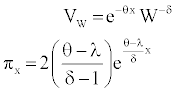(22)

From the above we can conclude that mean reversion can also be justified in the context of this model if δ>1, (φ<0) and θ>λ.

So we have arrived at the following proposition:

UProposition: UIn the context of the above model there exist parameter values, which are compatible with resolving the volatility puzzle, the equity premium puzzle and mean reversion simultaneously.

#### The Implications of the Model

Empirical studies (Ferson and Merrick , Schwert , Ferson and Harvey , Fama and French , and Kandel and Stambaugh  among others) have established that there exists a cyclical pattern in the temporal behavior of asset returns, the cyclicality being related to the business cycle. We therefore proposed here a model where investors behave cyclically. This is captured by a state dependent preference structure where a state variable is correlated with the market portfolio cum business cycle. In these circumstances, we prove that a negative correlation structure becomes instrumental towards explaining the three major puzzles. A negative correlation structure clearly implies that people are more relaxed in making economic decisions when the economy is booming but become more desperate when the economy is in recession. Of course with any concave utility function, the marginal utility is low in a boom and high in recession. But with the negative correlation structure we propose this effect becomes magnified.

Obviously, when agents exhibit such a cyclical preference structure, the temporal behavior of asset returns becomes cyclical.

It is possible to offer an intuitive explanation to the above interrelated phenomena. Consider investors at the thought of the business cycle expecting a rise. Since rises are highly persistent, the investor would expect the bull market to last for some time. One would also expect the marginal utility of consumption to fall and the demand for securities to rise along with security prices. Contrast this with investors at the peak anticipating a fall in the economic activity. One would expect that marginal utility of consumption would rise causing a shift away from securities and thus price declines. The point is that this cyclical change in the attitude should magnify swings in stock returns over the business cycle. The ultimate roots of swings in stock returns relate back to swings in economic activity in general. Hence mean reversion.

We also have high volatility in the stock market, not necessarily because the market is crowded with noise traders, but because investors have cyclically volatile preferences. In the face of a linear budget constraint and a concave, with respect to consumption, preference structure, investors still want to smooth out their consumption stream. But with the proposed preference structure, they will be affected by a cyclically volatile state variable. The correlation structure in our model allows this kind of transformation of the state variable volatility into the stock market volatility. In other words, a volatile state variable in the utility function becomes absorbed into the stock market and makes it volatile. Since the state variable in the preferences co-varies with the stock market, the consumption process does not have to move together with the stock market; consumption becomes smoother, and the stock market becomes more volatile.

The high equity premium is also justified within our model. When the stock market becomes more volatile (i.e., stock becomes more risky), the risk-free asset becomes more valuable since the safe asset provides a hedging service. When the safe asset becomes more valuable, the real interest rate becomes lower. The observed high equity premium can now be explained through this low real interest rate as data also confirms.

#### Conclusion

We have introduced a model that is built around a state dependent preference structure where the state dependency is related to the business cycle. In this setting the volatility puzzle, the equity premium puzzle and mean-reversion appear to be interrelated phenomena.

We have also identified the state variable in the preference structure in terms of its correlation structure with the market portfolio (business cycle). So investors behave cyclically in our model. But how can we interpret this state variable in the preference structure? We argue below that it is due to a liquidity-related imperfection.

Many economists have argued that liquidity-related imperfections follow the business cycle, and that the failure of the standard model comes from this liquidity-related imperfection . Moreover, Mehra and Prescott  argue that an alternative preference structure must be shown useful for organizing and interpreting not only behaviors in the financial markets but also those in other markets. If we interpret this alternative preference structure as something related to liquidity, then the extension to other markets follows easily. Liquidity-related imperfections are well recognized in terms of other markets, and many economists argue that economic phenomena can be better explained by incorporating this liquidity-related imperfection (for an earlier survey of the literature see Hayashi ).

Indeed the state dependency of our model can be thought of as a Lucas  taste shock to the utility function, which could, in turn, approximate a decision problem in the face of liquidity constraints. Liquidity constraints are difficult to deal with since they imply multilinear or non-linear budget constraints. According to the Household Production Approach  though, we can effectively deal with state dependent preference structure with a single linear budget constraint, by interpreting the state variable as something related to liquidity, say, a liquidity need. Our model, therefore, can be thought of as an attempt to capture the flavor of liquidity constraints, and yet avoid the analytical intractability of modeling them directly while working within the context of the standard model. The question of empirically testing the proposed model is left to future research.

#### Acknowledgements

The authors are grateful to William Brock, George Constantinides, Duffie , Bong-Soo Lee, Howard Thompson and John B. Donaldson for comments while they retain responsibility for any remaining errors. Christos Giannikos wishes to acknowledge a summer research grant from the Wasserman Department of Economics and Finance, Zicklin School of Business, Baruch College, CUNY.

#### References

Select your language of interest to view the total content in your interested language

### Article Usage

• Total views: 12262
• [From(publication date):
December-2013 - Feb 27, 2020]
• Breakdown by view type
• HTML page views : 8482Can't read the image? click here to refresh